# Examining Numerical Data¶

Last time, as part of a very broad intro to the class, we learned that data comes in two main flavors:

• Numerical
• Categorical

Today, we'll take a closer look numerical data. We'll see some of the computer code that we can use to wrangle it and we'll see some of the formulae that quantify it.

This is mostly covered in section 2.1 of our text.

## CDC Data¶

Let's start with a specific, real world data set obtained from the Center for Disease Control that publishes loads of data - the Behavioral Risk Factor Surveillance System.

This is an ongoing process where over 400,000 US adults are interviewed every year. The resulting data file has over 2000 variables ranging from simple descriptors like age and weight, through basic behaviors like activity level and whether the subject smokes to what kind of medical care the subject receives.

I've got a subset of this data on my website listing just 8 variables for a random sample of 20000 individuals: https://www.marksmath.org/data/cdc.csv

Our sample of the CDC data set is a bit more than 1Mb; it's bet to view it programmatically. Here's how to load and view a bit of it in Python:

import pandas as pd
df.tail()

genhlth exerany hlthplan smoke100 height weight wtdesire age gender
19995 good 1 1 0 66 215 140 23 f
19996 excellent 0 1 0 73 200 185 35 m
19997 poor 0 1 0 65 216 150 57 f
19998 good 1 1 0 67 165 165 81 f
19999 good 1 1 1 69 170 165 83 m

Most of the variables (ie., the column names) are self-explanatory. My favorite is smoke100, which is a boolean flag indicating whether or not the individual has smoked 100 cigarettes or more throughout their life. You should probably be able to classify the rest as numerical or categorical.

### Describing one dimensional, numerical data¶

Pandas provides a simple way to describe a list of numerical data. Here's a description of the heights in the CDC data, for example:

h = df['height']
h.describe()

count    20000.000000
mean        67.182900
std          4.125954
min         48.000000
25%         64.000000
50%         67.000000
75%         70.000000
max         93.000000
Name: height, dtype: float64

## Visualizing one dimensional, numerical data¶

In the previous slide, we see the following min, max and percentiles, which can be visualized using a box plot.

min 25% 50% 75% max
48 64 67 70 93
df.boxplot('height', vert=False, grid=False);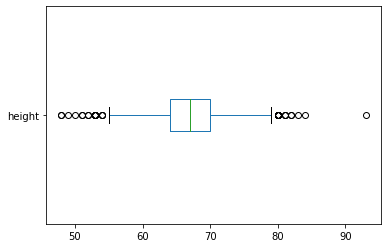### Ignoring outliers¶

Sometimes,we might want to ignore outliers

df.boxplot('height', vert=False, grid=False, showfliers=False);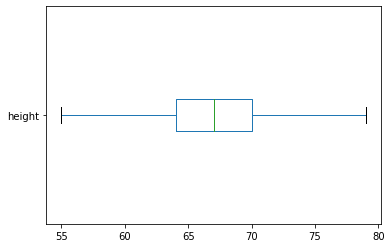### Histograms¶

A histogram provides a different picture of the data.

h.hist(bins = 20, grid=False, edgecolor='black');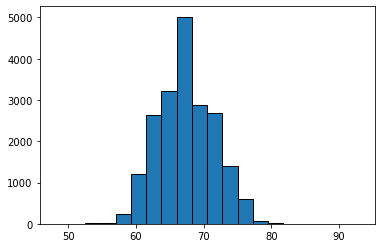### The mean for a histogram¶

While a box plot is tied to the min, max, and quantiles of the data, a histogram is tied to the mean and standard deviation of the data. It's fairly easy to see how the mean fits into normally distributed data like this:

import matplotlib.pyplot as plt
h.hist(bins = 20, grid=False, edgecolor='black');
m = h.mean()
print('m =',m)
plt.plot([m,m],[0,5100], 'y--');

m = 67.1829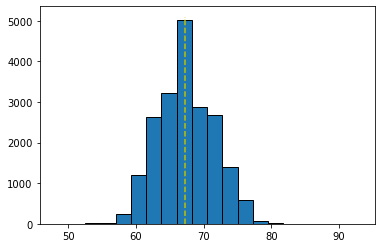### Standard deviation and histograms¶

To see the effect of standard deviation you really need to compare two or more histograms, as shown here. The code for this picture is a bit more involved so I've suppressed it.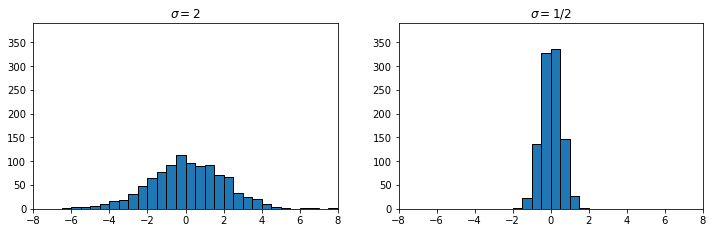You can experiment with the effect of mean and standard deviation in the interactive demo below.

### Other distributions¶

It's worth mentioning that there are other types of distributions that can arise.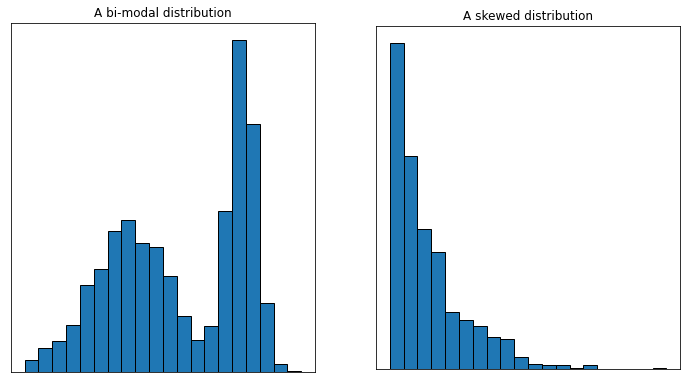### Scatter plots for 2D data¶

Finally, we sometimes need to visualize the relationship between variables. The ideal way to do that is with a scatter plot. For example, here's the relationship between height and weight in the CDC data.

df.plot('height', 'weight', 'scatter',
c=[(0.1,0.1,0.8,0.2)]
);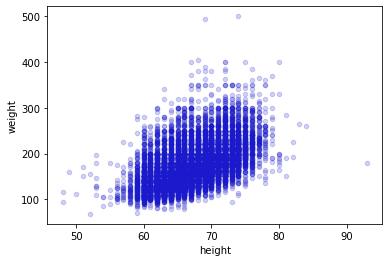### CFB¶

Correlating wins and losses to stats in College Football

## Definitions¶

At this point we've met several parameters that describe numerical data, including

• The mean,
• The median,
• percentiles, and
• the standard deviation

Let's take a look at how these quantities are actually defined.

Before we go through these, it's worth pointing out that the mean and standard deviation are the most important to understand thoroughly.

It's worth understanding percentiles from a conceptual standpoint, but we will rarely compute them directly. We will compute mean and standard deviation.

### The mean¶

The mean is a measure of where the data is centered. It is computed by simply averaging the numbers.

For example, our data might be $$2, 8, 2, 4, 7.$$ The mean of the data is then $$\frac{2+8+2+4+7}{5} = \frac{23}{5} = 4.6.$$

### The median¶

Like the mean, the median is a measure of where the data is centered.

Roughly speaking, it represents the middle value. They way it is computed depends on how many numbers are in your list.

If the number of terms in your data is odd, then the median is simply the middle entry.

For example, if the data is $$1,3,4,8,9,$$ then the median is $4$.

If the number of terms in your data is even, then the median is simply the average of the middle two entries.

For example, if the data is $$1,3,8,9,$$ then the median is $(3+8)/2 = 5.5$.

### Percentiles (also called quantiles)¶

• The median is a special case of a percentile - 50% of the population lies below the median and 50% lies above.
• Similarly, 25% of the population lies below the first quartile and 75% lies above.
• Also, 75% of the population lies below the third quartile and 25% lies above.
• The second quartile is just another name for the median.
• The inter-quartile range is the difference between the third and first quartile.
• One reasonable definition of an outlier is a data point that lies more than 3 inter-quartile ranges from the median.

#### Example¶

Suppose our data is $$4, 5, 9, 7, 6, 10, 2, 1, 5.$$ To find percentiles, it helps to sort the data: $$1,2,4,5,5,6,7,9,10.$$

• The median is definitely 5,
• the $25^{\text{th}}$ percentile might be 4,
• the $75^{\text{th}}$ percentile could be 7,
• and the inter-quartile range would be 3.

There are differing conventions on how you interpolate when the number of terms doesn't work well with the percentile, but these differences diminish with sample size.

### Variance and standard deviation¶

• The interquartile range forms a measure of the spread of a population or sample related to the median of that population or sample.
• The standard deviation forms a measure of the spread of a population or sample related to the mean of the population or sample.
• Roughly, the standard deviation measures how far the individuals deviate from the mean on average.
• The variance is defined to be the square of the standard deviation. Thus, if the standard deviation is $s$, then the variance is $s^2$.

### Definitions¶

• If we have a sample of $n$ observations $$x_1,x_2,x_3, \ldots, x_n,$$ then the variance is defined by $$s^2 = \frac{(x_1 - \bar{x})^2 + (x_2-\bar{x})^2 +\cdots+(x_n-\bar{x})^2}{n-1}.$$
• If $s^2$ is the variance, then $s$ is the standard deviation.

### Example¶

Suppose our sample is $$1,2,3,4.$$ Then, the mean is $2.5$ and the variance is $$s^2=\frac{(-3/2)^2 + (-1/2)^2 + (1/2)^2 + (3/2)^2}{3} = \frac{5}{3}.$$ The standard deviation is $$s = \sqrt{5/3} \approx 1.290994.$$

### Sample variance vs population variance¶

• You might see the definition $$s^2 = \frac{(x_1 - \bar{x})^2 + (x_2-\bar{x})^2 +\cdots+(x_n-\bar{x})^2}{n}.$$
• The difference in the definition is the $n$ in the denominator, rather than $n-1$.
• The difference arises because
• The definition with the $n$ in the denominator is applied to populations and
• The definition with the $n-1$ in the denominator is applied to samples.
• To make things clear, we will sometimes refer to sample variance vs population variance.

More often than not, we will be computing sample variance and the corresponding standard deviation.

### A quick problem¶

Suppose we have the data set

$$-1,4,3,4.$$

What are the mean and standard deviation?